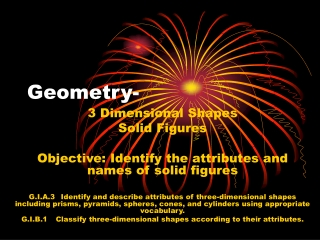# Geometry- - PowerPoint PPT PresentationDownload PresentationGeometry-

Geometry-
Download Presentation## Geometry-

- - - - - - - - - - - - - - - - - - - - - - - - - - - E N D - - - - - - - - - - - - - - - - - - - - - - - - - - -
##### Presentation Transcript

1. 3 Dimensional Shapes Solid Figures Objective: Identify the attributes and names of solid figures G.I.A.3 Identify and describe attributes of three-dimensional shapes including prisms, pyramids, spheres, cones, and cylinders using appropriate vocabulary. G.I.B.1 Classify three-dimensional shapes according to their attributes. Geometry-

2. What are solid figures? Solid Figures are 3D. Solid Figures can have: Faces Edges Vertices

3. Face A face is the flat surface of a solid figure. Choose a shape that has a face. How many faces does your shape have? Bonus: Does a sphere have any faces?

4. Edges An edge is formed when two faces meet. An edge is a straight line segment. Choose a shape that has an edge. How many edges does your shape have? Does a cone have an edge?

5. Vertices A vertex is a point where edges meet. Choose a shape that has a vertex. How many vertices does your shape have? Does a cylinder have a vertex?

6. Examples of Solid Figures

7. Cube Examples

8. Rectangular Prism Examples

9. Sphere Examples

10. Cone Examples

11. Cylinder Examples

12. Pyramid Examples

13. Hemisphere Examples

14. Review What is a solid figure? 1. flat, closed, plane figure; can’t stand on its own. 2. 3-D; it can stand on its own 3. None of the above

15. What is this figure called? 1. Cone 2. Rectangular Prism 3. Cube

16. What is this figure called? 1. Sphere 2. Cube 3. Pyramid

17. What is this part of the cylinder called? 1. Face 2. Edge 3. Vertex

18. What is this part of the cone called? 1. Face 2. Edge 3. Vertex

19. What is this part of the cube called? 1. Face 2. Edge 3. Vertex